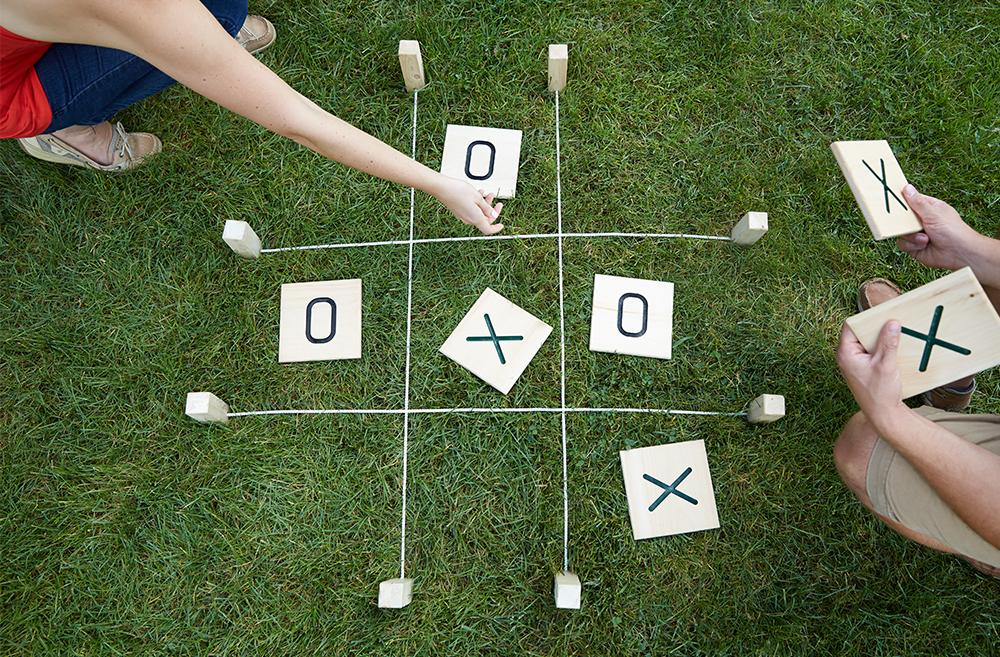Milo Yip：用C++编写游戏容易吗？有什么开源的小游戏吗？能分享一下吗？ 这个答案中，我提到学习游戏编程可从回合制游戏开始，例如井字棋。

## 1. 游戏状态的表示

``````typedef struct {
int board;    // -1 = empty, 0 = O, 1 = X
int turn;           // O first
} state;

void init(state* s) {
int i, j;
for (j = 0; j < 3; j++)
for (i = 0; i < 3; i++)
s->board[j][i] = -1;
s->turn = 0;
}``````

## 2. 显示游戏状态

`````` 1 | 2 | 3
---+---+---
4 | 5 | 6
---+---+---
7 | 8 | 9 ``````

``````void display(const state* s) {
int i, j;
for (j = 0; j < 3; j++) {
for (i = 0; i < 3; i++) {
switch (s->board[j][i]) {
case -1: printf(" %d ", j * 3 + i + 1); break;
case  0: printf(" O "); break;
case  1: printf(" X "); break;
}
if (i < 2)
printf("|");
else
printf("\n");
}
if (j < 2)
printf("---+---+---\n");
else
printf("\n");
}
}``````

``````void display(const state* s) {
int i, j;
for (j = 0; j < 3; printf(++j < 3 ? "---+---+---\n" : "\n"))
for (i = 0; i < 3; putchar("||\n"[i++]))
printf(" %c ", s->board[j][i] == -1 ? '1' + j * 3 + i : "OX"[s->board[j][i]]);
}``````

``````int main() {
state s;
init(&s);
display(&s);
} ``````

## 3. 实现下棋

``````int move(state* s, int i, int j) {
if (s->board[j][i] != -1)
return 0;
s->board[j][i] = s->turn++ % 2;
return 1;
}``````

``````int main() {
state s;
init(&s);
display(&s);
move(&s, 1, 1);
display(&s);
move(&s, 0, 1);
display(&s);
}``````

## 4. 处理输入

``````void human(state* s) {
char c;
do {
printf("%c: ", "OX"[s->turn % 2]);
c = getchar();
while (getchar() != '\n');
printf("\n");
} while (c < '1' || c > '9' || !move(s, (c - '1') % 3, (c - '1') / 3));
}``````

``````int main() {
state s;
init(&s);
display(&s);
while (s.turn < 9) {
human(&s);
display(&s);
}
}``````

## 5. 胜负判定

``````#define CHECK(j1, i1, j2, i2, j3, i3) \
if (s->board[j1][i1] != -1 && s->board[j1][i1] == s->board[j2][i2] && s->board[j1][i1] == s->board[j3][i3]) \
return s->board[j1][i1] == 0 ? 1 : -1;

int evaluate(const state* s) {
int i;
for (i = 0; i < 3; i++) {
CHECK(i, 0, i, 1, i, 2);    // horizontal
CHECK(0, i, 1, i, 2, i);    // vertical
}
CHECK(0, 0, 1, 1, 2, 2);        // diagonal
CHECK(0, 2, 1, 1, 2, 0);        // diagonal
return 0;
}``````

``````int main() {
state s;
init(&s);
display(&s);
while (s.turn < 9) {
human(&s);
display(&s);
switch (evaluate(&s)) {
case  1: printf("O win\n"); return 0;
case -1: printf("X win\n"); return 0;
}
}
printf("Draw\n");
}``````